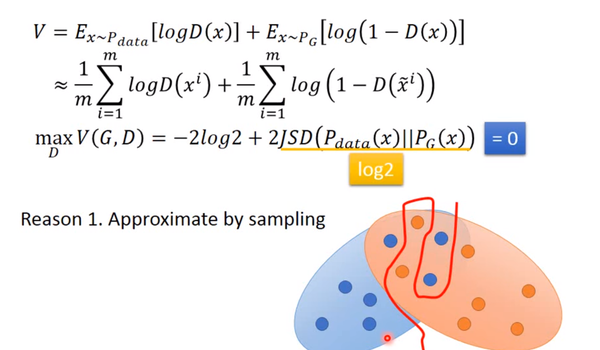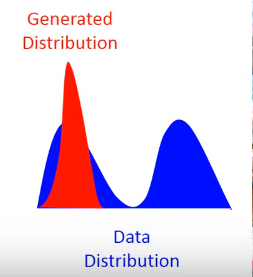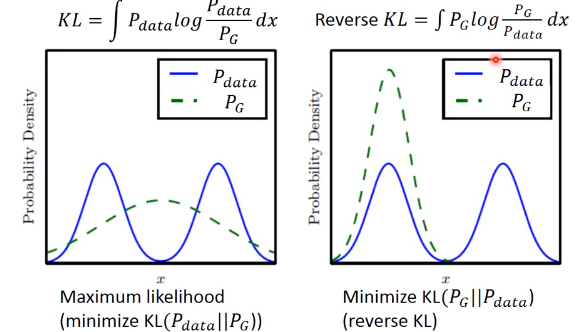# GAN原理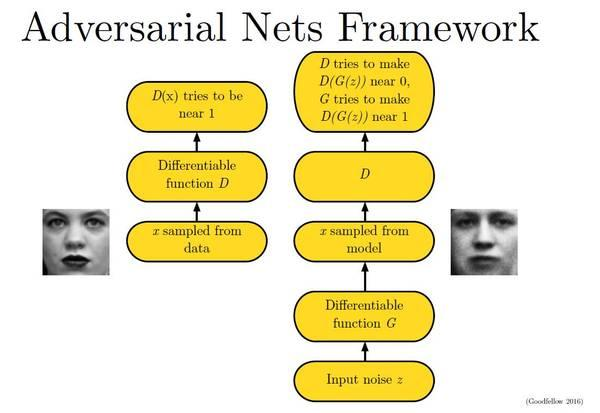## 1.Generation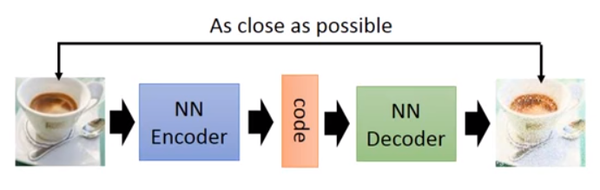## 2.GAN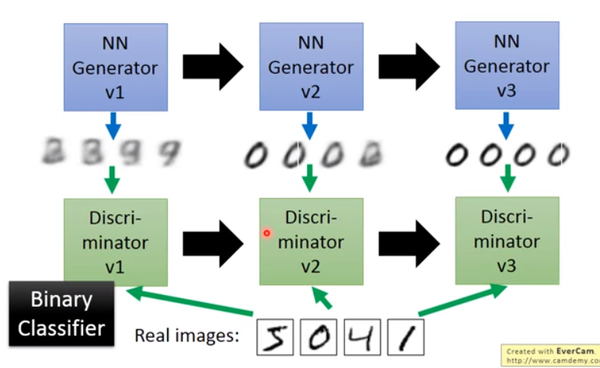## 3.原理图 1.26.1.1 - \begin{align} \theta ^\* &= arg\ \max\_{\theta}\prod\_{i=1}^{m}P\_G$$x^i;\theta$$ \\ &=arg\ \max\_{\theta}\ log\prod\_{i=1}^{m}P\_G$$x^i;\theta$$ \\ &=arg\ \max\_{\theta} \sum\_{i=1}^{m}logP\_G$$x^i;\theta$$ \\ & \approx arg\ \max\_{\theta}\ E\_{x\sim P\_{data}}$logP\_G$$x;\theta$$$ \\ & = arg\ \max\_{\theta}\int\_{x} P\_{data}$$x$$logP\_G$$x;\theta$$dx - \int\_{x}P\_{data}$$x$$logP\_{data}$$x$$dx \\ &=arg\ \max\_{\theta}\int\_{x}P\_{data}$$x$$$$logP\_G\(x;\theta$$-logP\_{data}$$x$$\)dx \\ &=arg\ \min\_{\theta}\int\_{x}P\_{data}$$x$$log \frac{P\_{data}$$x$$}{P\_G$$x;\theta$$}dx \\ &=arg\ \min\_{\theta}\ KL$$P\_{data}\(x$$\|\|P\_G$$x;\theta$$\) \end{align}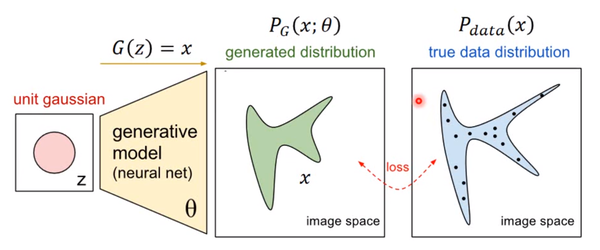图 1.26.1.2 - V$$G,D$$=E\_{x\sim P\_{data}}$logD$$x$$$ + E\_{x\sim P\_G}$log$$1-D\(x$$\)$图 1.26.1.3 - G^\*=arg\ \min\_{G}\ \max\_D\ V$$G,D$$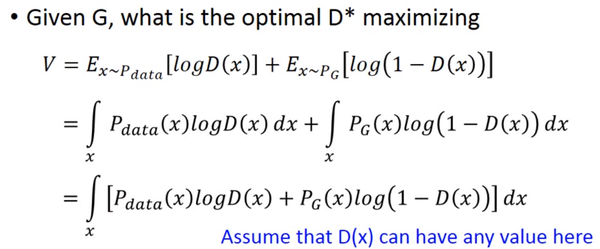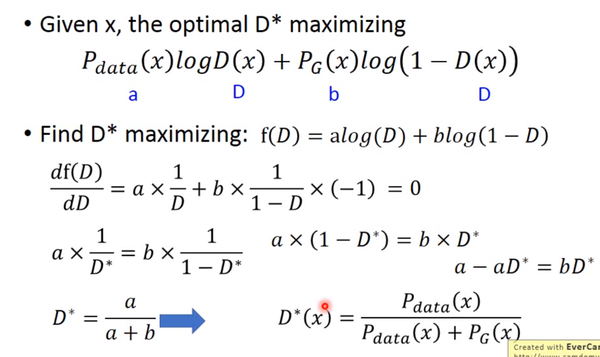对于一个给定的x，得到最优的D如上图，范围在(0,1)内，把最优的D带入，可以得到：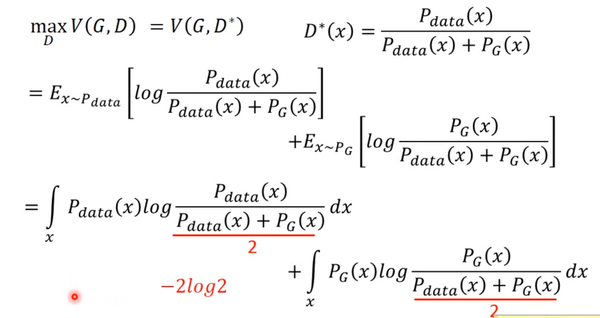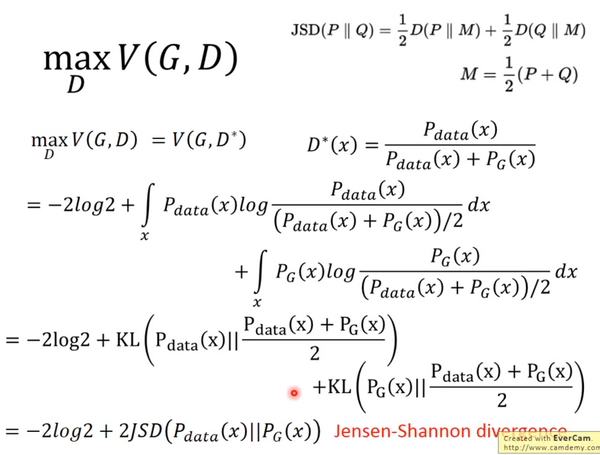JS divergence是KL divergence的对称平滑版本，表示了两个分布之间的差异，这个推导就表明了上面所说的，固定G，表示两个分布之间的差异，最小值是-2log2，最大值为0。

## 4.训练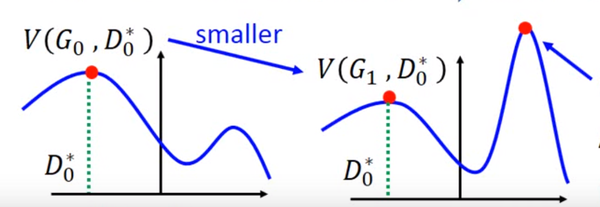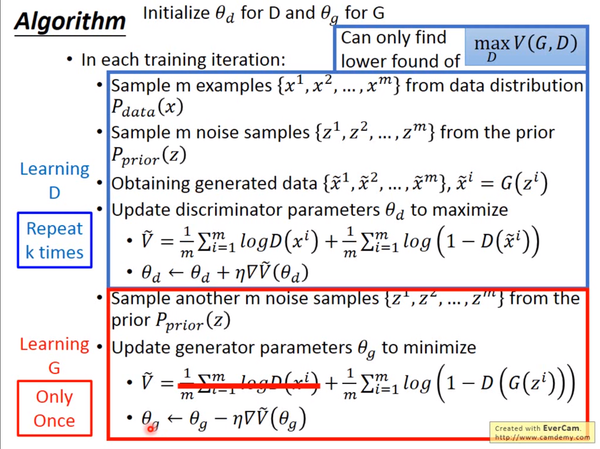## 5.存在的问题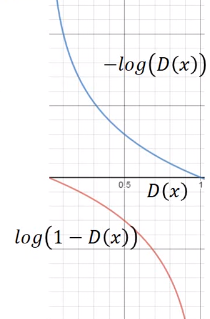是我们计算时G的loss function，但是我们发现，在D(x)接近于0的时候，这个函数十分平滑，梯度非常的小。这就会导致，在训练的初期，G想要骗过D，变化十分的缓慢，而上面的函数，趋势和下面的是一样的，都是递减的。但是它的优势是在D(x)接近0的时候，梯度很大，有利于训练，在D(x)越来越大之后，梯度减小，这也很符合实际，在初期应该训练速度更快，到后期速度减慢。